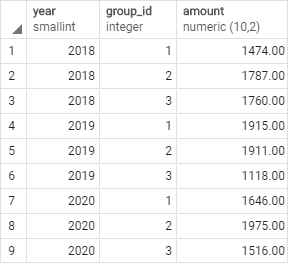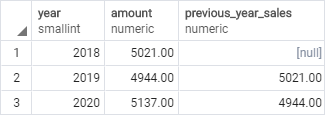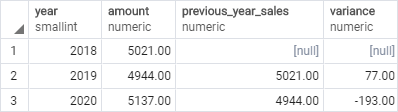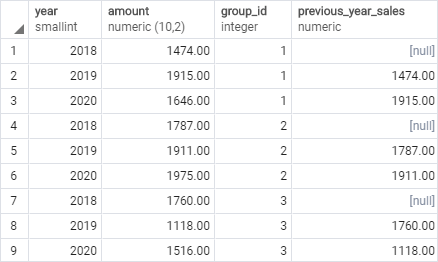# PostgreSQL Tutorial: LAG Function

August 7, 2023

Summary: in this tutorial, you will learn how to use the PostgreSQL `LAG()` function to access a row which comes before the current row at a specific physical offset.

## Introduction to PostgreSQL `LAG()` function

PostgreSQL `LAG()` function provides access to a row that comes before the current row at a specified physical offset. In other words, from the current row the `LAG()` function can access data of the previous row, or the row before the previous row, and so on.

The `LAG()` function will be very useful for comparing the values of the current and the previous row.

The following shows the syntax of `LAG()` function:

``````LAG(expression [,offset [,default_value]])
OVER (
[PARTITION BY partition_expression, ... ]
ORDER BY sort_expression [ASC | DESC], ...
)
``````

In this syntax:

### `expression`

The `expression` is evaluated against the row that comes before the current row at a specified offset. It can be a column, expression, or subquery. The `expression` must return a single value, and cannot be a window function.

### `offset`

The `offset` is a positive integer that specifies the number of rows which comes before the current row from which to access data. The `offset` can be an expression, subquery, or column. It defaults to 1 if you don’t specify it.

### `default_value`

The `LAG()` function will return the `default_value` in case the `offset` goes beyond the scope of the partition. The function will return NULL if you omit the `default_value`.

### PARTITION BY clause

The `PARTITION BY` clause divides rows into partitions to which the `LAG()` function is applied.

By default the function will treat the whole result set as a single partition if you omit the `PARTITION BY` clause.

### ORDER BY clause

The `ORDER BY` clause specifies the order of the rows in each partition to which the `LAG()` function is applied.

## PostgreSQL `LAG()` function examples

We’ll use the `sales` table from the `LEAD()` function tutorial for the demonstration.

Here is the data from the `sales` table:### 1) Using PostgreSQL `LAG()` function over a result set example

This example uses the `LAG()` function to return the sales amount of the current year and the previous year:

``````WITH cte AS (
SELECT
year,
SUM(amount) amount
FROM sales
GROUP BY year
ORDER BY year
)
SELECT
year,
amount,
LAG(amount,1) OVER (
ORDER BY year
) previous_year_sales
FROM
cte;
``````

Here is the output:In this example:

• First, the CTE returns net sales summarized by year.
• Then, the outer query uses the `LAG()` function to return the sales of the previous year for each row. The first row has NULL in the `previous_year_sales` column because there is no previous year of the first row.

This example uses two common table expressions to return the sales variance between the current and previous years:

``````WITH cte AS (
SELECT
year,
SUM(amount) amount
FROM sales
GROUP BY year
ORDER BY year
), cte2 AS (
SELECT
year,
amount,
LAG(amount,1) OVER (
ORDER BY year
) previous_year_sales
FROM
cte
)
SELECT
year,
amount,
previous_year_sales,
(previous_year_sales - amount) variance
FROM
cte2;
``````### 2) Using PostgreSQL `LAG()` function over a partition example

This example uses the `LAG()` function to compare the sales of the current year with the sales of the previous year of each product group:

``````SELECT
year,
amount,
group_id,
LAG(amount,1) OVER (
PARTITION BY group_id
ORDER BY year
) previous_year_sales
FROM
sales;
``````

This picture shows the output:In this example:

• The `PARTITION BY` clause distributes rows into product groups (or partitions) specified by group id.
• The `ORDER BY` clause sorts rows in each product group by years in ascending order.
• The `LAG()` function is applied to each partition to return the sales of the previous year.

In this tutorial, you have learned how to use the PostgreSQL `LAG()` function to access a row that comes before the current row at a specific physical offset.